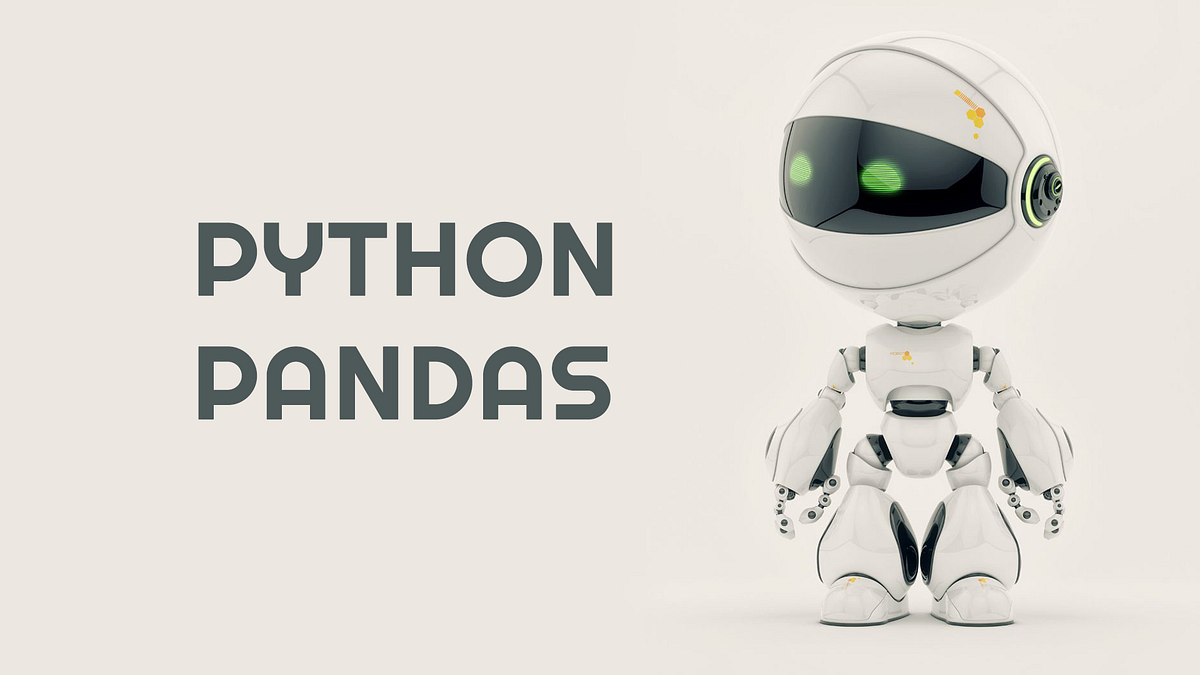# PYTHON Pandas Basic FunctionsPYTHON Pandas Basic Functions. So far, we have learned the three pandas data structure and how to create them. Due to its importance in real-time data processing, we will focus on dataframe objects right now and mention a few other data structures.

So far, we have learned the three pandas data structure and how to create them. Due to its importance in real-time data processing, we will focus on dataframe objects right now and mention a few other data structures.

## Basic Functions of Series

• axes: Returns the list of row axis tags.
• *dtype: *Returns the dtype object.
• empty: Returns false in serial empty job.
• *ndim: *Returns the number of dimensions of the underlying data as definition 1.
• *size: *Returns the number of basic data items.
• *values: *Returns the series as ndarray.
• *head: *Returns the first n lines.
• *tail: *Returns the last n lines.

Let’s consolidate the above structures with examples.

Example 1

``````import pandas as pd
import numpy as np

s = pd.Series(np.random.randn(4))
print(s)``````

0 1.373370 1 -0.960120 2 0.143523 3 0.553266 dtype: float64

``````
**Example 2 (axes)**

Returns the list of row axis tags.
``````

import pandas as pd import numpy as np

``````s = pd.Series(np.random.randn(4))
print(s.axes)``````

`````` [RangeIndex(start=0, stop=4, step=1)] ```

## Data Science Course in Dallas

Become a data analysis expert using the R programming language in this [data science](https://360digitmg.com/usa/data-science-using-python-and-r-programming-in-dallas "data science") certification training in Dallas, TX. You will master data...

## Python for Data Science - Course for Beginners (Learn Python, Pandas)

This Python data science course will take you from knowing nothing about Python to coding and analyzing data with Python using tools like Pandas, NumPy, and ...

## Python for Data Science - Data Science with Python - Python Training

This Edureka video on "Python For Data Science" explains the fundamental concepts of data science using python. It will also help you to analyze, manipulate, and implement machine learning using various python libraries such as NumPy, Pandas, and Scikit-learn.

## 50 Data Science Jobs That Opened Just Last Week

Data Science and Analytics market evolves to adapt to the constantly changing economic and business environments. Our latest survey report suggests that as the overall Data Science and Analytics market evolves to adapt to the constantly changing economic and business environments, data scientists and AI practitioners should be aware of the skills and tools that the broader community is working on. A good grip in these skills will further help data science enthusiasts to get the best jobs that various industries in their data science functions are offering.

## Data Science Projects | Data Science | Machine Learning | Python

Practice your skills in Data Science with Python, by learning and then trying all these hands-on, interactive projects, that I have posted for you.